## Systems of Linear Equations

#### Introduction

Consider the two equations ax+by=c and dx+ey=f. Since these equations represent two lines in the xy-plane, the simultaneous solution of these two equations (i.e. those points (x,y) that satisfy both equations) is merely the intersection of the two lines. The graphs below illustrate the three possible cases: non-parallel lines, parallel (but not identical) lines, and identical lines.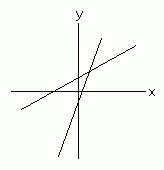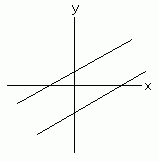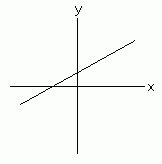From left to right these cases yield one solution, no solutions, and infinite solutions. The same situation occurs in three dimensions; the solution of 3 equations with 3 unknowns is the intersection of the 3 planes.

There is a simple tool for determining the number of solutions of a square system of equations: the determinant.

#### Matrix Notation

It is often convenient to represent a system of equations as a matrix equation or even as a single matrix. Consider the system of equations 2x+3y=-8 and -x+5y=1. Since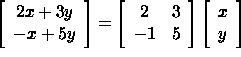we may write the entire system as a matrix equation: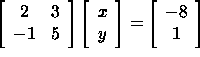or as AX=B where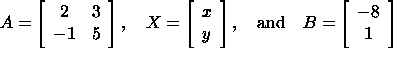The 2x2 matrix A is called the matrix of coefficients of the system of equations. Frequently this equation is written as a single augmented matrix: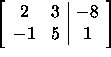We may now use Gaussian elimination to solve this matrix equation for x and y (as opposed to direct substitution of one equation into the other). This representation can also be done for any number of equations with any number of unknowns.

In general, the equation AX=B representing a system of equations is called homogeneous if B is the nx1 (column) vector of zeros. Otherwise, the equation is called nonhomogeneous.

#### Determining the Number of Solutions of a Nonhomogeneous System of Equations

For square systems of equations (i.e. those with an equal number of equations and unknowns), the most powerful tool for determining the number of solutions the system has is the determinant. Suppose we have two equations and two unknowns: ax+by=c and dx+ey=f with b and e non-zero (i.e. the system is nonhomogeneous). These are two lines with slope -a/b and -d/e, respectively. Let's define the determinant of a 2x2 system of linear equations to be the determinant of the matrix of coefficients A of the system. For this system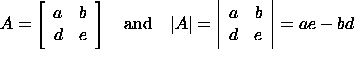Suppose this determinant is zero. Then, this last equation implies a/b=d/e; in other words, the slopes are equal. From our discussion above, this means the lines are either identical (there is an infinite number of solutions) or parallel (there are no solutions). If the determinant is non-zero, then the slopes must be different and the lines must intersect in exactly one point. This leads us to the following result:

A nxn nonhomogeneous system of linear equations has a unique non-trivial solution if and only if its determinant is non-zero. If this determinant is zero, then the system has either no nontrivial solutions or an infinite number of solutions.

#### Example

The nonhomogeneous system of equations 2x+3y=-8 and -x+5y=1 has determinant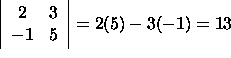which implies the system has a unique solution (the point (-43/13,-28/75)). This corresponds to our intuition: the lines have different slopes and therefore must intersect in exactly one point.

#### Example

The nonhomogeneous system of equations x-4y+6z=3, -2x+8y-12z=-6, and  2x-y+3z=1 does not have a unique solution since its determinant is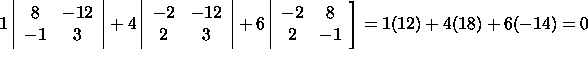In fact, this system has an infinite number of solutions. To see this, think geometrically. The three equations represent 3 planes. Two of the equations (the first and the second) represent the same plane (why?); so, there are only two distinct non-parallel planes. Since two non-parallel planes intersect in a line, there are an infinite number of points which lie on all three of these planes (i.e. the system has an infinite number of solutions). To actually find the solutions, we must use a method such as Gaussian elimination to solve the system of equations.

#### Determining the Number of Solutions of a Homogeneous System of Equations

For a homogeneous system of equations ax+by=0 and cx+dy=0, the situation is slightly different. These lines pass through the origin. Thus, there is always at least one solution, the point (0,0). If the slopes -a/b and -c/d are equal then there are an infinite number of solutions since the lines are identical. But as we have seen, the slopes of these lines are equal when the determinant of the coefficient matrix is zero. Thus, for homogeneous systems we have the following result:

A nxn homogeneous system of linear equations has a unique solution (the trivial solution) if and only if its determinant is non-zero. If this determinant is zero, then the system has an infinite number of solutions.

#### Example

The system of equations 2x+y=0 and x-y=0 has exactly one solution since the slopes of the lines are different, i.e. the determinant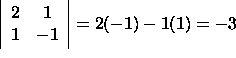is non-zero. The solution is of course (0,0).